# Team:NEU CHINA/Model

NEU_CHINAModel

Model is a way to simulate and predict the genetic circuits we design using a mathematical or software-based tool. This is a very important part of our program. Our models can help us in the following ways.
-More concise to show the genetic circuits we designed.
-Better understand the mechanism of action of the system.
-Estimate the parameters of the biochemical reactions involved in the system.
-Compare with data from wet lab to predict the result of wet lab, which is instructive.
-May reflect issues that we had not noticed and optimize our design of the project.Background

The design of our project is based on AHL-induced quorum sensing (QS) system to amplify the detection effect of engineered bacteria on viruses. The engineered bacteria bind to the virus through the PmrCAB system, and this signal will cause LuxI gene expression, promote AHL production and bind to the expression product of LuxR gene to promote the expression of downstream gene circuit reporter proteins. In addition, the AHL produced by the engineered bacteria can spread to the extracellular medium and enter into other engineered bacteria that are not bound to the virus to promote the expression of their downstream reporter genes, thus achieve a high sensitivity effect.

Our model is mainly based on the mass action law, which reflects the rate of biochemical reactions such as transcription, translation and degradation, and Hill's law, which simulates the synergistic nature of ligand binding; by comparing with the data and results of wet lab, our model predicted results fit the data of wet lab well and provide a reference for prediction of subsequent experiments.

Based on the design of our project, we constructed four models: AHL production and release, AHL-based quorum sensing system, application of Hrp amplifier, and improvement of PLux_R promoter. These models show the progress of various biochemical reactions in our project. During the modeling process we made various assumptions by referring to the literature and combining them with the actual situation of our project, and also to narrate the problem more concisely and reduce the workload. We have omitted many trivial details based on the assumptions to put more emphasis on the design features of our project, and without affecting the predictions of the model itself for the project.

Biochemical

For the QS circuit, the main biochemical reactions can be split in two main classes: the gene expression reactions, and the induction ones.
For the gene expression part, the key processes and assumptions for the genes LuxI, LuxR and EGFP are:
-The binding of RNA polymerase (RNAP) to each promoter is a fast reaction, therefore it has not been evaluated.
-Binding of transcription factors to gene promoters.
-Degradation of mRNA, transcription factors and proteins.
In the part of induction based on the self-inducing agent AHL, the main processes considered are: Design of hetero- and homodimerization reactions of inducers, such as the binding of proteins LuxR and AHL to form a monomer, which dimerizes to form a dimer
-Diffusion of inducers through the cell membrane.
-Binding of dimer to PLux_R, PLux_HS (a modification of the PLux_R promoter).
-Degradation of monomers, dimers and inducers.
These biochemical species react according to the following assumptions:
1. During transcription, the cell contains enough free RNAP to serve all the active genes transcribing at a given moment. In this way, it is assumed that the free RNAP concentration in the cell will not appreciably change in time,
2. Basal expression or leakiness (i.e. transcription even in saturating presence of the repressor) of the repressible promoter PLux_R is a nonzero minimal expression level,
3. Translation is not a simple process. It was modeled as an irreversible reaction with an average transcription rate accounting for the fact that binding of ribosomes to the ribosome binding site (RBS) is indeed reversible, and several ribosomes may translate a single messenger RNA copy (mRNA) simultaneously,
4. Transcription of genes LuxR, LuxI, and EGFP is irreversible, so that ktrc-R , ktrc-I and ktrc-E are the effective transcription rates of LuxR, LuxI, and EGFP respectively,
5. gR, gI, and gG are the plasmid copy numbers of genes LuxR, LuxI, and EGFP respectively,
6. LuxR and AHL binding is a fast and reversible reaction,
7. Dimerization of (LuxR. AHL)2 or (R.A)2 is a reversible reaction,
8. Interactions between AHL and AHLext represent the physical passive diffusion process for cell-to-cell communication via quorum sensing, so that was modeled as a reversible pseudo-reaction,
9. Vc = Vcell/Vext is the ratio between the cellular and the environment volumes to quantify the AHL/AHLext effect,
10. The monomer (LuxR. AHL) or (R.A) only degrades at each cell cycle,
11. Messengers RNA, proteins and transcription factor degradation are irreversible reactions (denoted as ∅).
Sender: We define sender as an engineered bacterium in a group of engineered bacteria that can bind to the virus surface antigen and can produce AHL first after a series of biochemical reactions. The generated AHL can stimulate downstream gene circuits to produce green fluorescent protein (EGFP) as a reporter protein. And the generated AHL can diffuse outside the cell for the engineered bacteria that are not bound to antigen to produce fluorescent signals.
Receptor: A group of engineered bacteria that are not able to bind to the antigen and need to first receive the AHL diffused by Sender before they can stimulate the downstream gene circuit to produce EGFP .
Sender and receptor are the same engineered bacterium. The only distinction is whether or not they receive direct stimulation from exogenous antigen.

Model construction presupposes that

1. The copy size of the corresponding DNA in each engineered bacterium is 10, and the DNA is not degraded.
2. LuxR is an endogenous protein and the relative amount of LuxR is maintained at 300 until the artificially introduced gene is expressed.
Reaction：
1)Expression of LuxI/LuxR
DNA_LuxI -> mRNA_LuxI -> LuxI
DNA_LuxR -> mRNA_LuxR -> LuxR
Reaction rate:
vtranscription=Ktrc*[DNA]
vtranlation=Ktrl*[mRNA]
Related parameters:
ktrc-R => LuxR Transcription rate constant
ktrc-I => LuxI Transcription rate constant
ktrl-R => LuxR Translation rate constant
ktrl-I => LuxI Translation rate constant
[DNA] => Relative content of the corresponding DNA
[mRNA] => Relative content of the corresponding DNA

2)Degradation of mRNA and protein of LuxI/LuxR
mRNA_LuxI -> null
mRNA_LuxR -> null
LuxI -> null
LuxR -> null
Reaction rate:
Related parameters:
k deg-mI =>mRNA_LuxI Degradation rate constant
k deg-mR =>mRNA_LuxR Degradation rate constant
k deg-I =>LuxI Degradation rate constant
k deg-R =>LuxR Degradation rate constant
[Pr] => Relative content of the corresponding protein

3)Synthesis of AHL
Sender.LuxI -> Sender.AHL + Sender.LuxI
Reaction rate: vA=kAI(t)
Related parameters: kA=>AHL Synthesis rate; I(t)=>LuxI Concentration

Sender.AHL -> null
Receptor.AHL -> null
Reaction rate
Intracellular degradation rate: v=[k A-deg in]*H(t)
Extracellular degradation rate: v=[k A-deg out]*Hex(t)
Related parameters:
k A-deg in => Intracellular AHL degradation rate
k A-deg out => Extracellular AHL degradation rate

5)Diffusion of AHL
AHL（Intracellular）→AHL（Extracellular）（sender）
Reaction rate: v=r(H(t)-Hex(t))（H(t)>Hex(t)）
AHL（Intracellular）→AHL（Extracellular）（receptor）
Reaction rate: v=r(Hex(t)-H(t))（Hex(t)>H(t)）
Related parameters:
r=> Diffusion coefficient H(t)=>Intracellular AHL concentration Hex(t)=>Extracellular AHL concentration

Explanation: AHL diffusion is actually a relatively complex process. After referring to the projects of iGEM team in previous years, in order to simplify the problem, we regard the transmembrane diffusion process of AHL as simple free diffusion, which can be described by Fick's law, that is, the flux of transmembrane molecules is directly proportional to the gradient concentration. In our model, the transmembrane rate of AHL is directly proportional to the concentration difference on both sides.
In addition, we assume that the diffusion rate of AHL across the membrane is fast enough, ignoring the process of AHL propagation in the membrane, and AHL can reach a stable state in a short time in the culture medium and inside the cell, that is, AHL is evenly distributed in the system.

6)Combination of AHL and LuxR
AHL + LuxR [(R.A)]
Reaction rate:
υ=kf_1*AHL*LuxR - kr_1*[(R.A)]
Related parameters:
kf_1 => Rate for the combination of AHL and LuxI; The complexes are denoted as (R.A)
The complexes are denoted as (R.A)kr_1 => The dissociation rate constant of (R.A), is the rate for the combined inverse reaction of AHL and LuxI.
From the Hill equation and the law of mass action it follows that Binding rate/ Dissociation rate = dissociation constant, there is an equation between the three, and the value of the third quantity can be found by looking up the value of only two of them. The rate of the reaction can be simulated by knowing the binding rate and the dissociation.

7)(R.A) dimerization forms (R.A)2
[(R.A)] [(R.A)2]
Reaction rate:
v=kf_2*[(R.A)]*[(R.A)] - kr_2*[(R.A)2]
Related parameters:
kf_2 =>Rate for the synthesis of (R.A)2 by (R.A) dimerization
kr_2 =>Rate for dissociation of (R.A)2 into (R.A)

(R.A) -> null
(R.A)2 -> null
Reaction rate:
Related parameters:
k deg(R.A) => Degradation rate of (R.A)
k deg(R.A)2 => Degradation rate of (R.A)2
[(R.A)] => Relative content of (R.A)
[(R.A)2] => Relative content of (R.A)2

9)(R.A)2 binds to promoter PLux_HS
(R.A)2 + PLux_HS <-> [PLux_HS-(R.A)2]
Reaction rate:
v=kf_3*(R.A)2*PLux_HS - kr_3*PLux_HS-(R.A)2
Related parameters:
kf_3 => receptor Binding rate constant of (R.A)2 dimer to promoter PLux_HS in engineered bacteria
kf_s3 => sender Binding rate constant of (R.A)2 dimer to promoter PLux_HS in engineered bacteria
kr_3 => Dissociation rate constants for the separation of (R.A)2 dimer from the promoter PLux_HS
The number of copies of promoter PLux_HS in the model of this simulation is 10, then the number of formed PLux_HS-(R.A)2 cannot exceed the number of copies of promoter PLux_HS. So the number of events PLux_HS-(R.A)2 is 10 when it is set here.
According to literature, promoter PLux_HS needs to bind to the (R.A)2 dimer to drive downstream gene expression. However, when the concentration of (R.A)2 dimer is too high, the activity of promoter PLux_HS is inhibited instead. o setting the event assumes that the value of kf_3 changes from 10 to 0.01 when the amount of (R.A)2 exceeds 300, same with kf_s3.

10)Downstream gene circuit of promoter PLux_HS
EGFP fluorescent protein expression: DNA_EGFP -> mRNA_EGFP-> EGFP
Expression of LuxI：DNA_LuxI -> mRNA_LuxI -> LuxI
Related parameters:
ktrc-E => EGFP Transcription rate
ktrc-I => LuxI Transcription rate
ktrl-E => EGFP Translation rate
ktrl-I => LuxI Translation rate
EGFP is a reporter protein that can detect the presence and content of viruses in air and various liquids based on the intensity of this fluorescent signal.
LuxI can continue to produce AHL making further increases in AHL levels.
Increased levels of AHL accelerate the rate of expression of downstream gene circuits, and higher production of AHL enhances the effect of quorum sensing, allowing receptor-engineered bacteria to shine as quickly as possible.
Parameter

 Name description Value Constant kA-deg out Degradation rate of extracellular AHL 0.04 TRUE Kdeg-mR mRNA_LuxR degradation rate constant 0.247 TRUE k A AHL synthesis rate 0.04 TRUE kf_1 Rate constant of AHL and LuxI binding 1000 TRUE kf_2 Rate constant of dimerization synthesis of (R.A)2 20 TRUE k deg(R.A) (R.A) Degradation rate constant 0.156 TRUE ktrc-E EGFP transcription rate constant 0.8368 TRUE k deg-I LuxI degradation rate constant 3.09 TRUE k A-deg in Degradation rate of intracellular AHL 0.057 TRUE k deg(R.A)2 (R.A)2 Degradation rate constant 0.017 TRUE kdeg-mE mRNA_EGFP degradation rate constant 0.247 TRUE Kdeg-E EGFP degradation rate constant 0.027 TRUE r diffusion coefficient of AHL 3.4272 TRUE k deg-mI mRNA_LuxI degradation rate constant 0.247 TRUE Ktrl-I LuxI translation rate constant 2.9268 TRUE k degR LuxR degradation rate constant 0.2 TRUE kr_1 The dissociation rate constant of (R.A) 10 TRUE kr_2 (R.A)2 dissociates into the rate constant of (R.A) 1 TRUE kf_3 Binding rate constant of dimer (R.A)2 to promoter PLux_HS in engineered receptor bacteria 10 or 0.01 TRUE kr_3 Dissociation rate constant of (R.A)2 dimer from promoter PLux_HS 1 FALSE ktrc-R LuxR transcription rate constant 0.7968 TRUE ktrc-I LuxI transcription rate constant 0.9756 TRUE ktrl-R LuxR translation rate constant 2.3904 TRUE Ktrl-E EGFP translation rate constant 2.5104 TRUE kf_s3 The binding rate constant of (R.A)2 dimer to promoter PLux_HS in sender engineered bacteria 10 or 0.01 FALSE

We build the following four models based on the above responses and assumptions.

Model 1 AHL generation and release model

We found the release pattern of AHL under the effect of quorum sensing system of engineered bacteria by simulation results.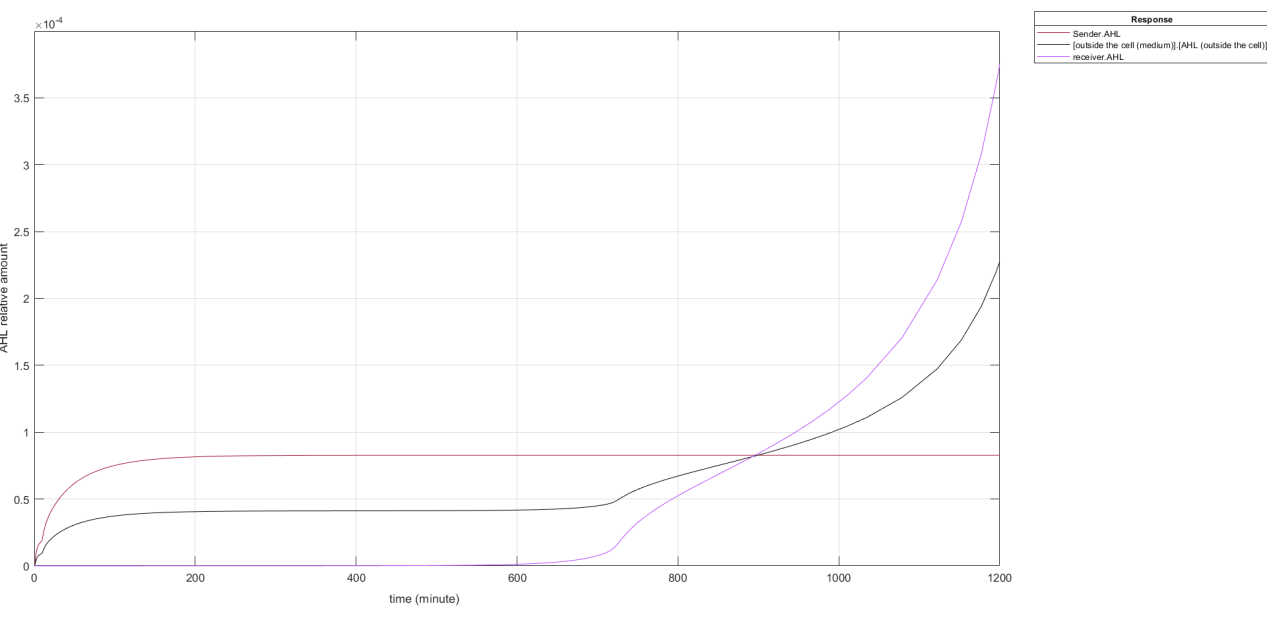Fig.1 Short-term change trends of AHL in the QS system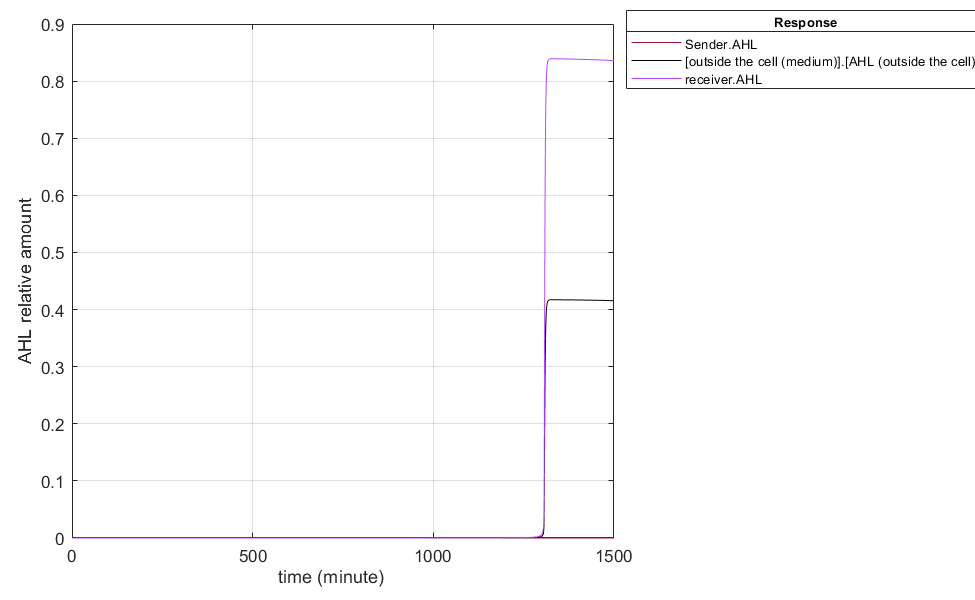Fig. 2 Long-term change trend of AHL in the QS system

Analysis of results:
According to the simulation graph we can see that the Sender produces AHL and reaches the balance around 180 min, which is the same trend as EGFP, only the difference between the relative contents. Receptor can only absorb AHL from the outside in the early stages, so its growth rate is very slow and almost non-existent. After reaching 600 min, the AHL content reached a certain threshold, causing the AHL in the receptor to bind to LuxR to stimulate the endogenous LuxI expression in the receptor and generate AHL before the content of AHL in the receptor increased rapidly. Since AHL enters and leaves the cell by free diffusion, the amount of extracellular AHL is always between the two, so the three lines will intersect at one point.

LuxR in Receptor is only endogenously supplied, and the promoter PLux_HS stimulation will not have LuxR continue to increase. Only the single pathway of endogenous LuxR gene supplies LuxR production, so there will be a decrease in LuxR content in the later stages, resulting in the promoter PLux_HS in the receptor not being continuously activated and a decrease in AHL content in the later stages of the receptor. As AHL crosses membranes in the form of free diffusion, the content of extracellular AHL decreases.

Model 2 Quorum Sensing System

We used the SimBiology app of Matlab2021a to simulate of our biochemical reaction processes, the following figure shows the model diagram we constructed based on the above responses.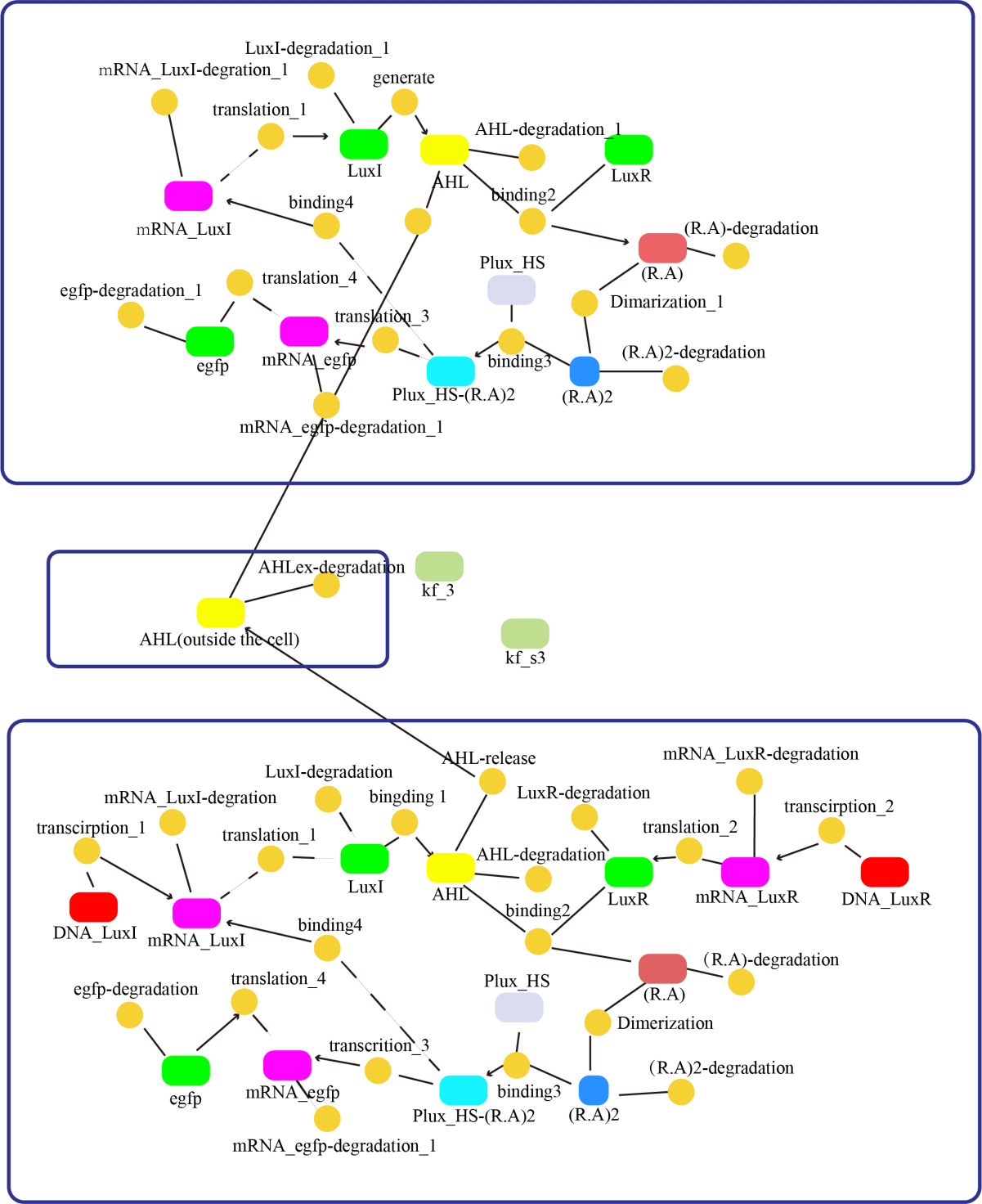Fig.3 Quorum sensing simulation chart

According to the short-term change trend of fluorescence relative content of the wet lab, we inferred that when the relative content of sender and receptor engineered bacteria is 1:3, it is closest to the experimental results. Through the model, we can infer the approximate time when the population induction takes effect, and can predict the later results of the wet lab, so that the length of monitoring time of the students in the we tlab group can be roughly expected. As in Figure 4 below.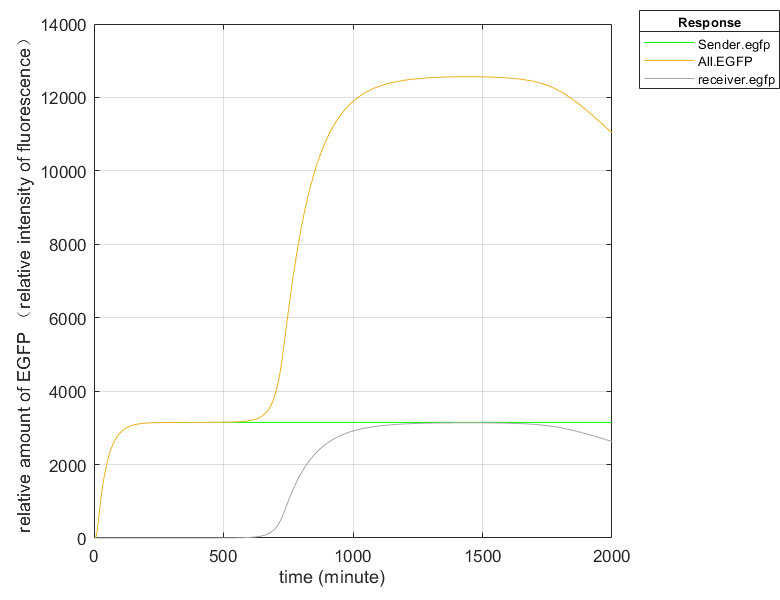Fig.4 EGFP content simulation graph (Fluorescence intensity simulation graph)

Results analysis:
When viruses were present in the environment, the Sender combined with the antigen on the virus surface to stimulate the expression of related genes and synthesize EGFP. Therefore, the fluorescence signal inside the Sender in the figure was detected first and reached equilibrium at about 180min. After that, AHL in the Sender accumulates to a certain extent, diffuses into the culture medium through free diffusion, and is absorbed by the Receiver, inducing the synthesis of EGFP, and also emits fluorescence, and the total fluorescence also increases synchronously. And since the relative number ratio of Sender and Receiver is 1:3, the fluorescence intensity at the final equilibrium is four times that of a single engineered bacterium.

Model 3 The application of Hrp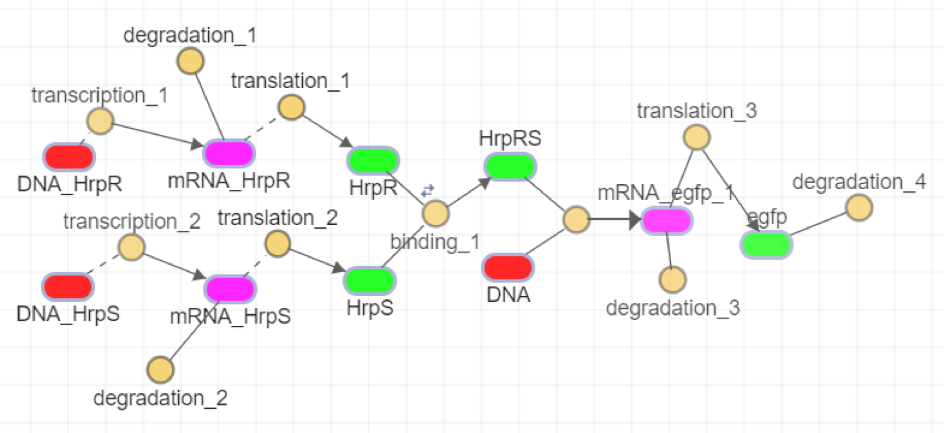Fig.5 Simulation chart of amplifier section

When we simulate using SimBiology in Matlab, PLux_HS-(R.A)2 has the same effect as DNA_HrpR and DNA_HrpS in Figure 5, and we substitute in the simulation to get the simulation results. As in Figure 6.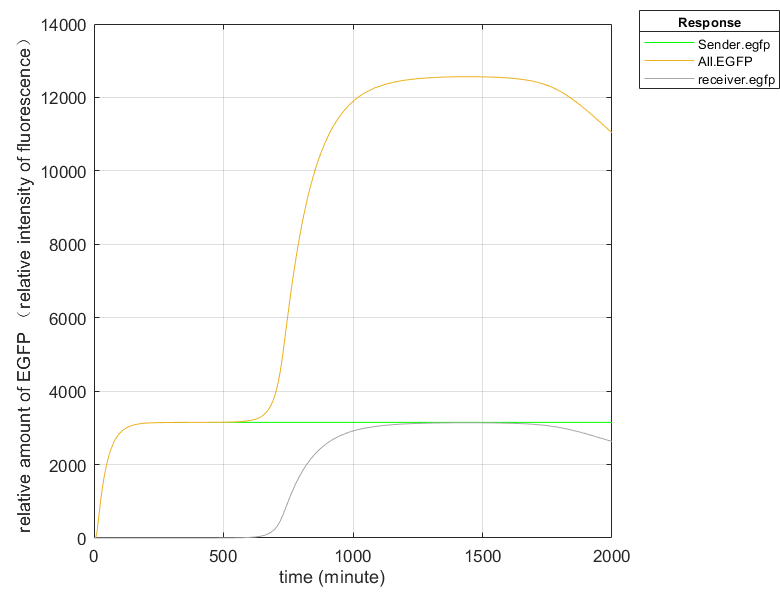Fig.6 (1) Fluorescence intensity plot without Hrp amplifier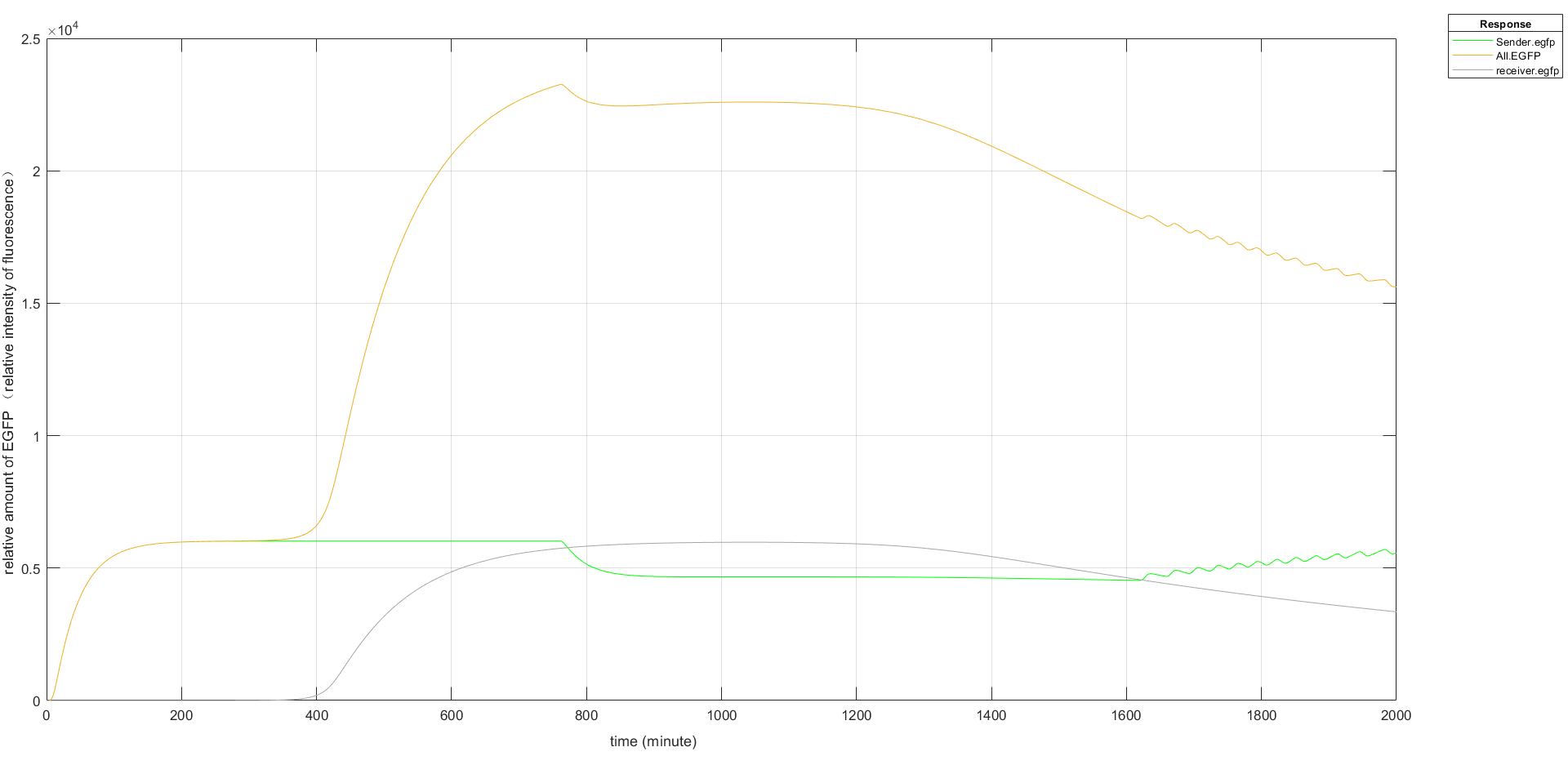Fig.6 (2) Fluorescence intensity plot with Hrp amplifier

In order to improve the expression effect of fluorescence, we used Hrp amplifier. Based on the simulation results, we can see that the expression of EGFP increases significantly after the addition of the amplifier, and the EGFP of Sender in Figure 6(2) oscillates up and down around a value due to the fact that (R.A) over 300 acts as a repressor for the promoter.

Model 4 Improvement of PLux_R promoter

The results of simulating promoter leakage through Matlab are plotted as follows.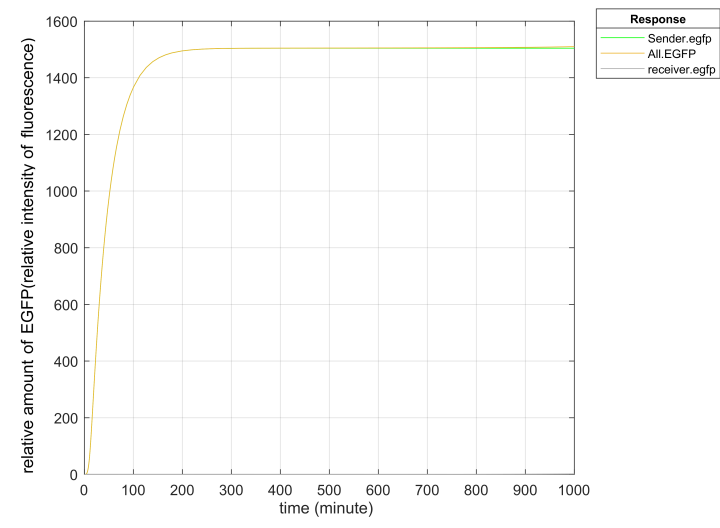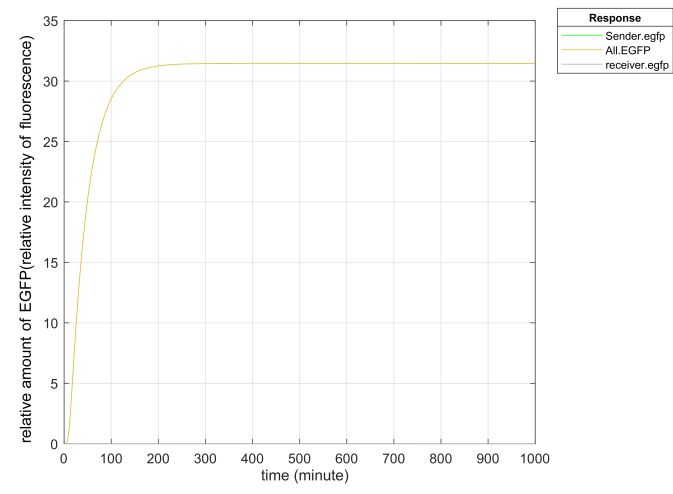Fig.7 (1) Simulation chart of PLux_R promoter     Fig.7 (2) Simulation chart of PLux_HS promoter

The PLux_HS we use has been improved by Tsinghua University and has low leakage. In the second gene circuit we designed, according to the stimulation, we found that the leakage of the PLux_R was far more than expected while the leakage rate of PLux_HS was about 1/50 of that of ordinary PLux_R promoter, and the leakage expression of EGFP of the improved promoter was far below the detection limit, which greatly reduced the basic expression of EGFP.

Reference

Chen, M., Liu, H., & Yan, F. (2019). Oscillatory dynamics mechanism induced by protein synthesis time delay in quorum-sensing system. Phys Rev E, 99(6-1), 062405.
 Landrain, T. E. , Carrera, J. , Kirov, B. , Rodrigo, G. , & Jaramillo, A. . (2009). Modular model-based design for heterologous bioproduction in bacteria. Current Opinion in Biotechnology, 20(3), 272-279.
 Zeeshan, S. , Christoph, K. , Heiko, B. , & D Mukesh. (2018). Mathematical modelling of ahl production in exiguobacterium mpo strain. Biochemical Engineering Journal, S1369703X18302183-.
 Weiss, J. N. . (1997). The hill equation revisited: uses and misuses. Faseb Journal, 11(11), 835-841.
 Meyer, A. , Megerle, J. A. , Kuttler, C. , Müller, Johannes, Aguilar, C. , & Eberl, L. , et al. (2012). Dynamics of ahl mediated quorum sensing under flow and non-flow conditions. Physical Biology, 9(2), 026007.
 Ripp, S. , Jegier, P. , Birmele, M. , Johnson, C. M. , Daumer, K. A. , & Garland, J. L. , et al. (2010). Linking bacteriophage infection to quorum sensing signalling and bioluminescent bioreporter monitoring for direct detection of bacterial agents. Journal of Applied Microbiology, 100(3), 488-499.